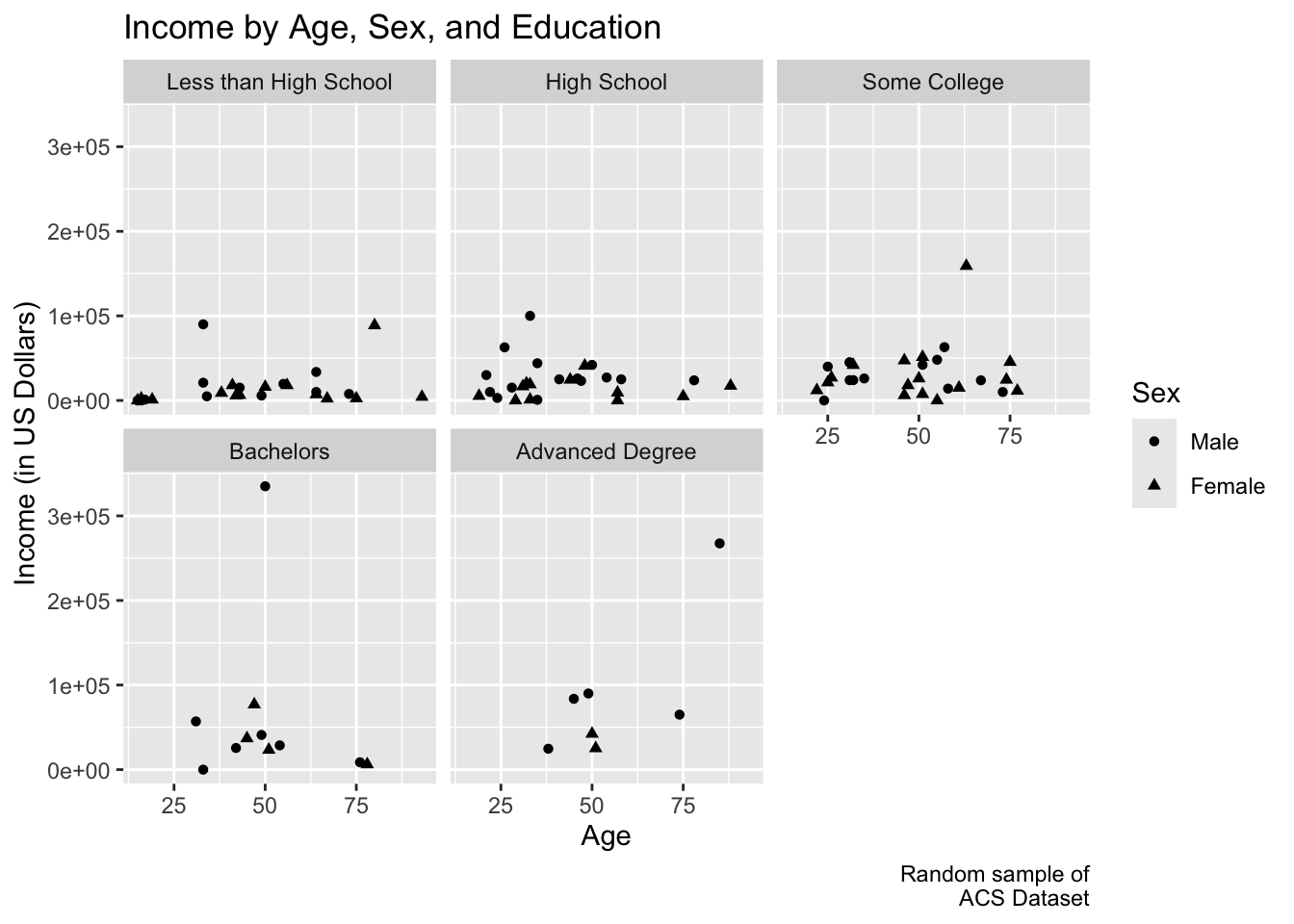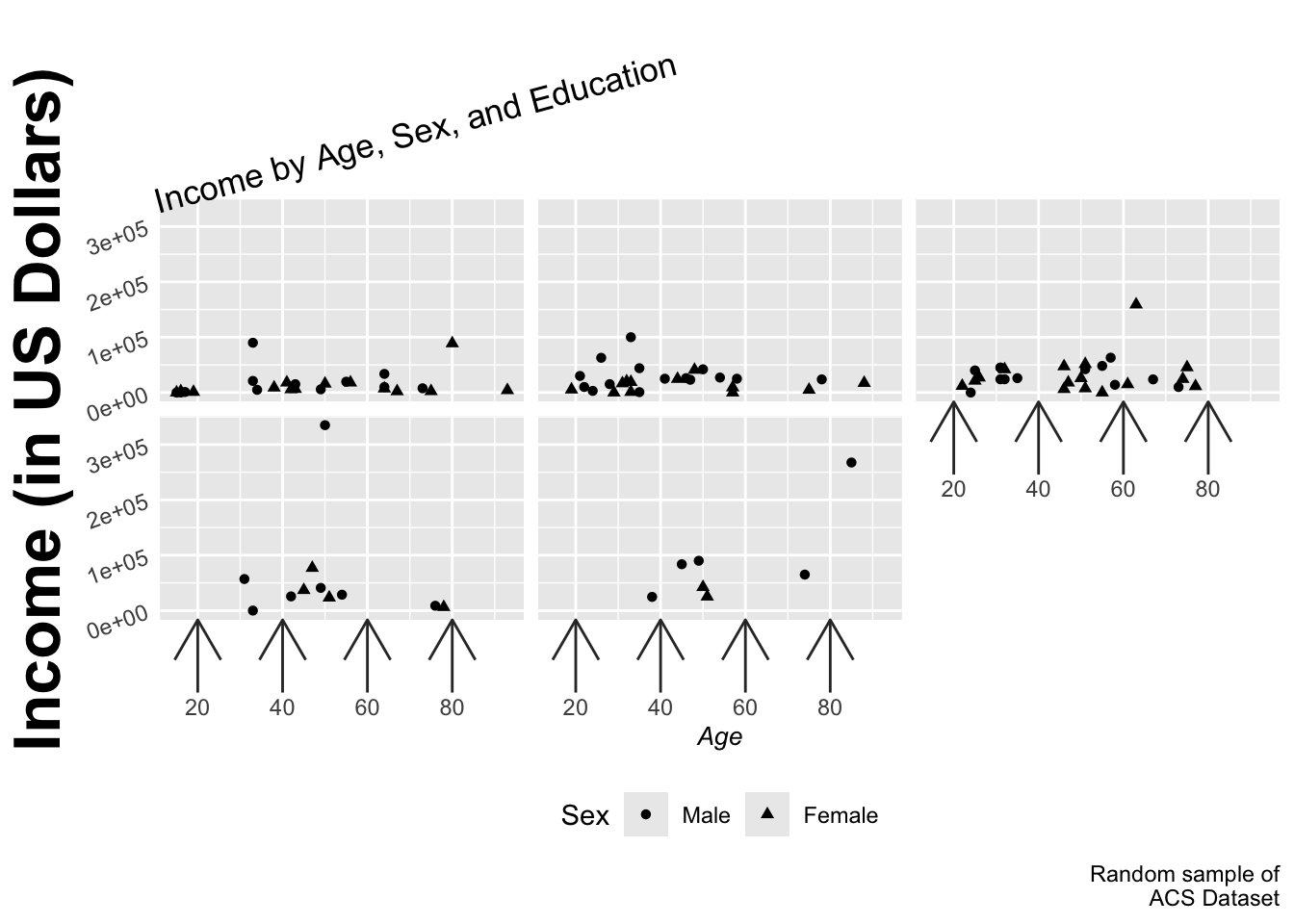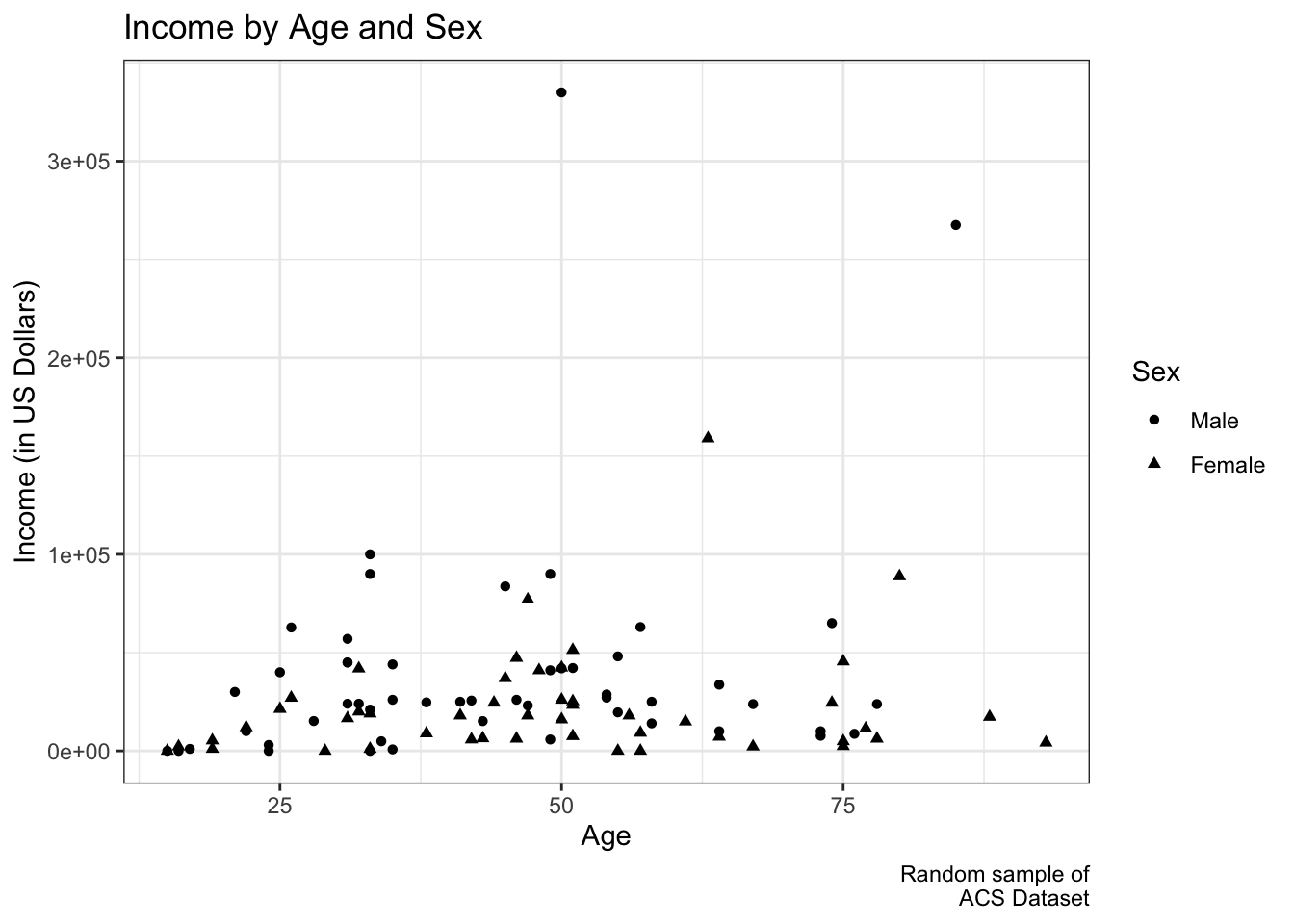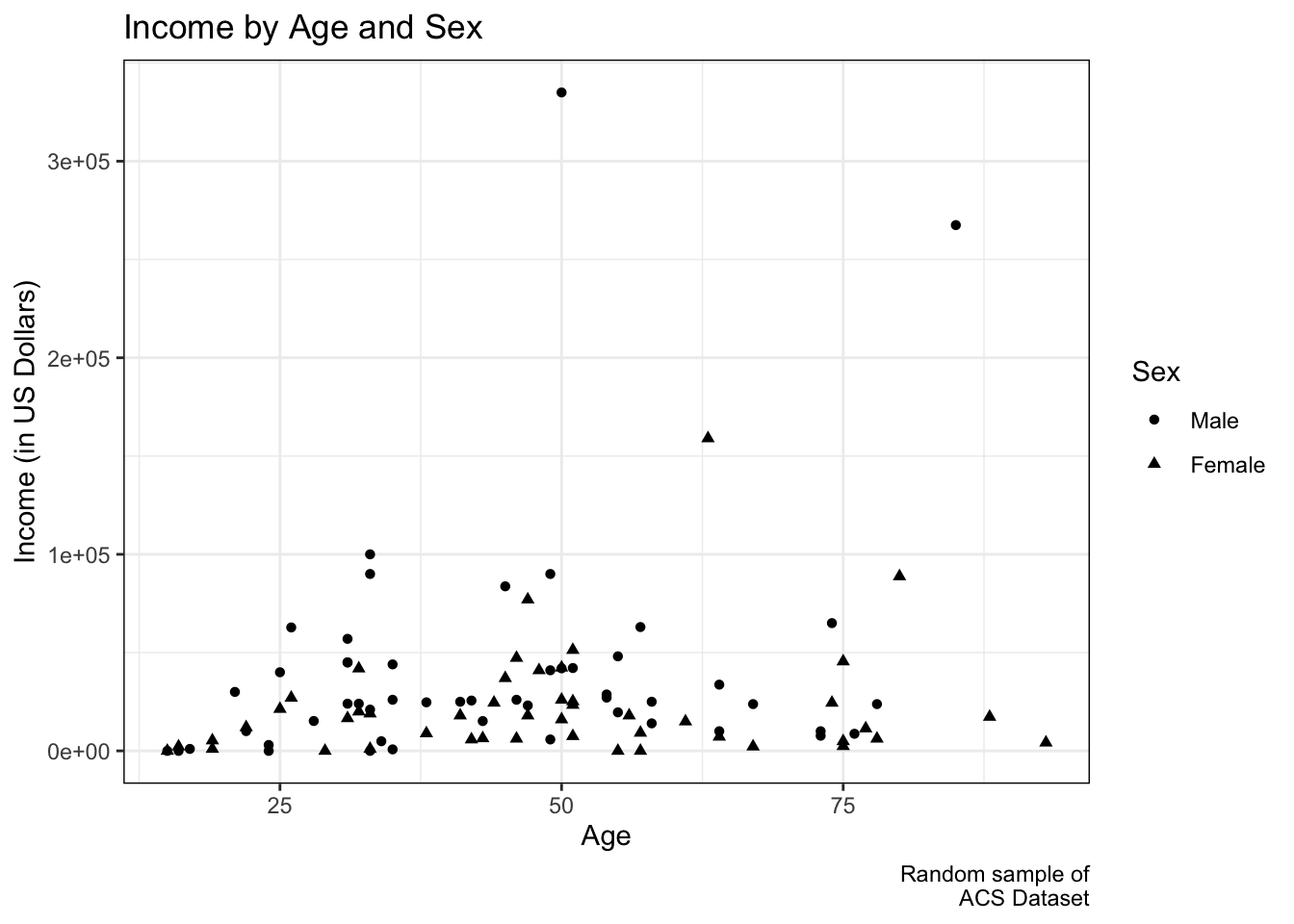# 8 Making Plots Pretty

If you are starting from this page, please run the code at Libraries and Data Setup before proceeding.

In the plots until this point, little attention was paid to making plots that look good, only making plots that we can understand.

ggplot has powerful functionality for customizing every bit of a plot, and with a bit of work, you can produce plots ready for presentations and publications.

## 8.1 Adding and Changing Labels

Labels can be added to a plot with `labs()` by assigning a character string to the aesthetic (x, y, shape, etc.). Setting labels is important for at least two reasons. First, we usually want to make our variable names look nice for presentations by capitlizing the first letters and clarifying meanings, like changing “income” to “Income (in US Dollars)”. Second, and more importantly, we want our plots to be interpretable. Unless a reader is already familiar with dummy coding in general and your dataset in particular, seeing “as.factor(female)” as a legend title and 0 and 1 as level labels will be confusing. To help others (including future-us) understand our plot more readily, we can call the variable “Sex” and its levels “Male” and “Female”.

``````ggplot(acs_sample, aes(x = age, y = income,
shape = as.factor(female))) +
geom_point() +
labs(title = "Income by Age, Sex, and Education",
caption = "Random sample of\nACS Dataset",
x = "Age",
y = "Income (in US Dollars)",
shape = "Sex") +
facet_wrap(~ edu) +
scale_shape_discrete(labels = c("Male", "Female"))``````## 8.2 Changing Text and Axes

Text size, font, angle, and position are all customizable. We can tell `theme()` what we would like to change. (To see a list of things that can be changed, see `help(theme)`.)

To remove something, we can set it equal to `element_blank()`. Below, the y-axis ticks and the facet labels were removed.

Axis breaks can be modified with one of the `scale_x_*()` or `scale_y_*()` arguments.

``````ggplot(acs_sample, aes(x = age, y = income,
shape = as.factor(female))) +
geom_point() +
labs(title = "Income by Age, Sex, and Education",
caption = "Random sample of\nACS Dataset",
x = "Age",
y = "Income (in US Dollars)",
shape = "Sex") +
facet_wrap(~ edu) +
scale_shape_discrete(labels = c("Male", "Female")) +
theme(axis.title.x = element_text(size = 10, face = "italic"),
axis.title.y = element_text(size = 25, face = "bold"),
axis.text.y = element_text(angle = 20),
axis.ticks.length.x = unit(1, "cm"),
axis.ticks.x = element_line(arrow = arrow()),
axis.ticks.y = element_blank(),
legend.position = "bottom",
plot.title = element_text(angle = 15, vjust = -.2),
strip.text = element_blank()) +
scale_x_continuous(breaks = seq(0, 100, 20))``````While this is certainly not a good plot, it hopefully gives you an idea of the customization capabilities of ggplot.

## 8.3 Changing Themes

Several preset themes are available in ggplot, including `theme_gray()` (default) and `theme_bw()` (shown below). (For a full list, see `help(theme_gray)`.) Many more themes are available in the `ggthemes` package, but you are free to customize everything (see `help(theme)`).

``````ggplot(acs_sample, aes(x = age, y = income,
shape = as.factor(female))) +
geom_point() +
labs(title = "Income by Age and Sex",
caption = "Random sample of\nACS Dataset",
x = "Age",
y = "Income (in US Dollars)",
shape = "Sex") +
scale_shape_discrete(labels = c("Male", "Female")) +
theme_bw()``````Or we can try to reproduce the above plot manually:

``````ggplot(acs_sample, aes(x = age, y = income,
shape = as.factor(female))) +
geom_point() +
labs(title = "Income by Age and Sex",
caption = "Random sample of\nACS Dataset",
x = "Age",
y = "Income (in US Dollars)",
shape = "Sex") +
scale_shape_discrete(labels = c("Male", "Female")) +
theme(panel.border = element_rect(color = "black", fill = NA),
panel.grid = element_line(color = "#EEEEEE"),
panel.background = element_rect(fill = NA),
legend.key = element_rect(fill = NA))``````After a while, you may create your own theme that you like very much. If you do so, you can start every script with assigning your preferences as a theme object. When making a plot, simply call that object, as done below with `myTheme`.

``````myTheme <- theme(panel.border = element_rect(color = "black", fill = NA),
panel.grid = element_line(color = "#EEEEEE"),
panel.background = element_rect(fill = NA),
legend.key = element_rect(fill = NA),
axis.title = element_text(size = 12),
axis.text.y = element_text(angle = 25))

acs %>%
filter(!is.na(race), !is.na(edu)) %>%
ggplot(aes(x = race, y = edu)) +
geom_jitter(size = .25, alpha = .1) +
labs(title = "Distribution of Race and Education",
caption = "Data from 2000 American Community Survey",
x = "Race",
y = "Education") +
myTheme``````